### Canvi idiomaxibec.jpeg
portada_05.jpg
collverd.jpeg
portada_03.jpg
cullerot.jpeg
portada_01.jpg
portada_06.jpg
gavina.jpeg
lluna.jpeg
mallarenga.jpeg
portada_02.jpg
xarrec.jpeg
portada_04.jpg# Register

To use the program dies to register as the program stores data (arrays) user to the server. Thus arrays will always be available. So when you access the program shows a window as in figure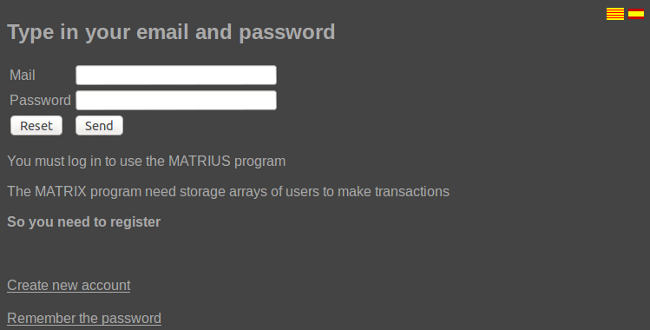ask for credentials.
At the top right you have the flags to change the language (available in English, Catalan and Spanish).
At the bottom we have a link to register if you have not we have not done and another to remember the password (send an email with the new password).

# Introduction.

Once we hagem accredited program shows a window like the figure:At the top of the window have the button to create a new array and import a CSV file.

On the right is a list of matrices that the user has saved. Each list item can be selected. You can select more than one array simultaneously.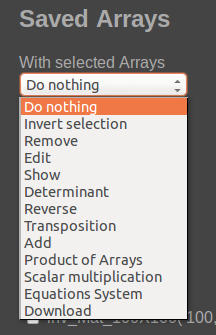We have a selection box as the figure to choose what we do with arrays indicated.
For normal operation of the program follow these steps:

• Select the array or matrix object of our operation.
• Select the operation of the combo box.
• On the left side of the window shows us the result of the operation.
• If the operation has been done without problems in the matrix resulting from the operation is stored in the list of arrays for later use.

# Create a new array

To create a new array to press the button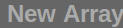in the upper left that shows a window like the figure:Enter the name and description of the new array. When we have introduced the number of rows and columns will appear in the table to enter the numbers as shown in the figure:Once you have typed the numbers and press the new array of arrays listed as shown in the figure:# Import a CSV file

To import a CSV file to press the button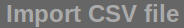at the top of the window. We work as a window of figure:You must select whether the column separator is a semicolon or a comma. You must give a name to the array, type the description and select the CSV file.
We can generate the CSV file manually or use a spreadsheet. If you use a spreadsheet should be noted that in cell A1 is having the value of the first row first column. In the spreadsheet should not have titles, there are only having values ​​in the array starting at cell A1.

# Remove matrices stored

To remove one or more arrays from the list you need to select the matrix or matrices to remove the combo box and select the Remove option.
On the left side of the window will list the arrays eliminated.

# Edit matrix

To edit the array must select the matrix edit in the matrix and select edit combo box. We work as a window of figure: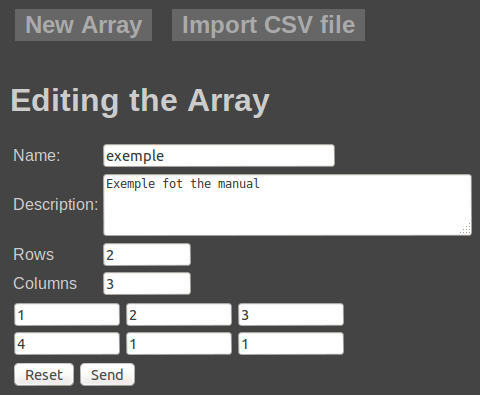We can edit the name, description and values ​​naturally.
If you modify the value of the rows or columns, with a smaller value and sends the data irreversibly lose the values ​​of the columns or rows deleted. Reducing the number of rows or columns can accidentally leave any editing option selected in the combo box but by pressing the Send button.

If we expand the number of columns or rows retain the values ​​of the original rows and columns.

# Show a matrix

We can show an array by clicking on the name in the matrix or by selecting it from the list and choose Show combo box. We see a window like the figure: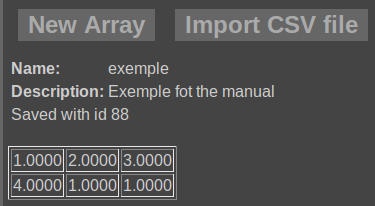We can select more than one array simultaneously and we show you selected as shown in the following figure: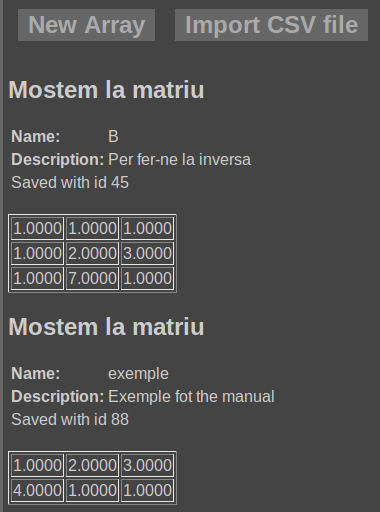# Calculate determinant

To calculate a determinant just select the array and combo box choose Determined. We are presented with a window like the figure: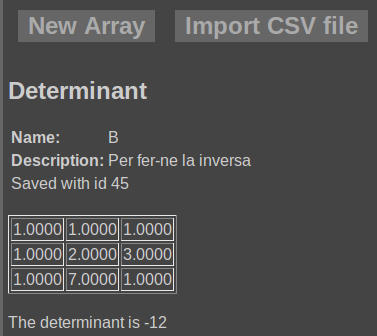Keep in mind that it is possible to calculate certain of square matrices.

# Calculate the inverse matrix

To calculate the inverse matrix simply select the combo box and choose Inverse. We are presented with a window like the figure.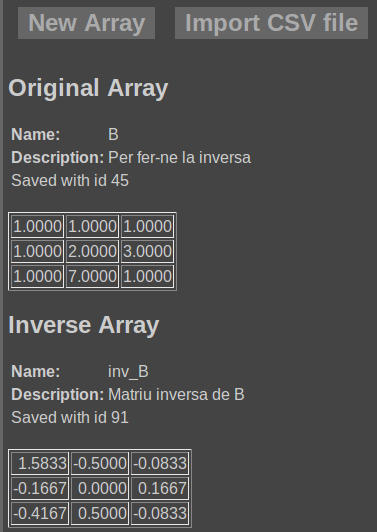To see a list of arrays with new array called Nommo inv_NomMat where is the name of the array we calculated the reverse as shown in the figure: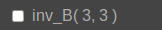You can only calculate inverse square matrices.

# Trasposta calculate a matrix

To calculate a matrix trasposta simply select it from the combo box and choose the option Trasposition. We see a window like the figure: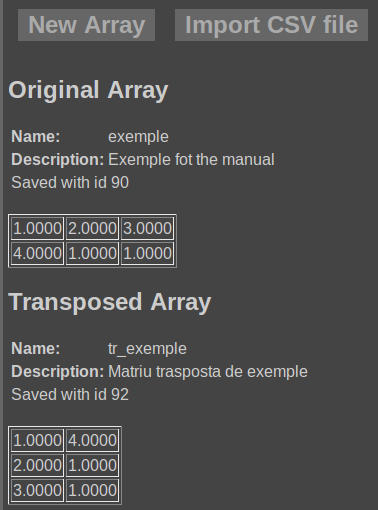To see a list of arrays with new array called Nommo tr_NomMat where is the name of the array that we trasposta calculated as shown in the figure: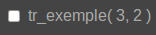# Adding arrays

To add two or more arrays must be selected for the combo box choose Add. We see a window like the figure: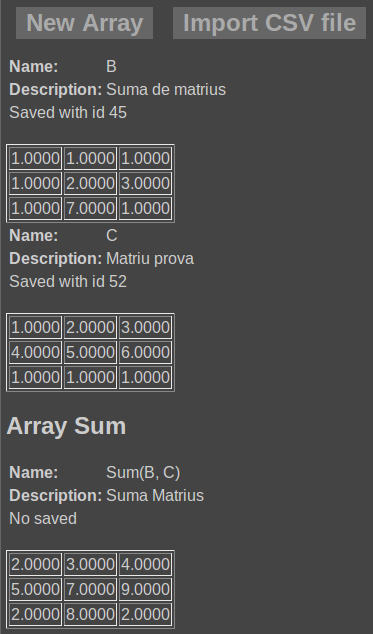To see a list of arrays with new array called Sum (Nom1, name2) where Nom1 and name2 are the names of the matrices that have joined.
To add two or more arrays must have the same number of rows and columns.

# Matrix multiplication

To compute the product of two matrices just select them and choose the combo product of arrays. If any of the products of the first matrix by the second, the second to the first or both the program computes and displays. To compute the product of two matrices should the number of columns in the first matrix is ​​the same as the number of rows of the second.
The program shows a window similar to the figure:The program saves the results calculated with the product name on Nom1 Nom1 * name2 is the name of the first array of bread products and name2 in the second.

# Scalar product

To calculate the scalar product of one or more arrays just select it or select it and the combo box to a scalar product. The program asks the scale as shown in the following figure: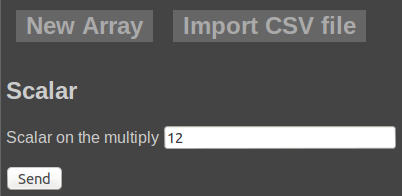By clicking the Send button the program shows the result as shown in the figure: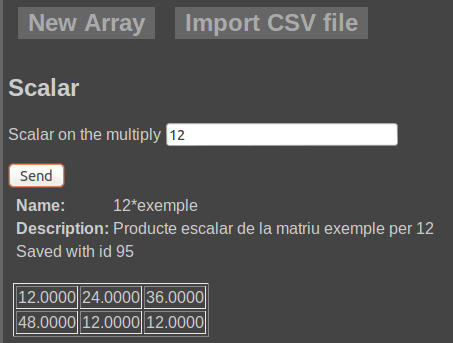The resulting matrix or matrices are stored with the name Nommo * Esc Esc on the scale and multiply that by the Nommo is the name of the array.

# Systems of equations

The program solved systems of equations whenever supported. The program interprets the matrix as follows
The first column, all but the last, the terms correspond to the questions multiply. Thus the first column corresponds to the first question, the second in the second ...
The last column corresponds to the term independent.
So the system prompt:

 3x + 2y + z= 12 x + y + z= 17 2x + 4y + 4z= 3

If we do that with this system we get the result shown in the following figure: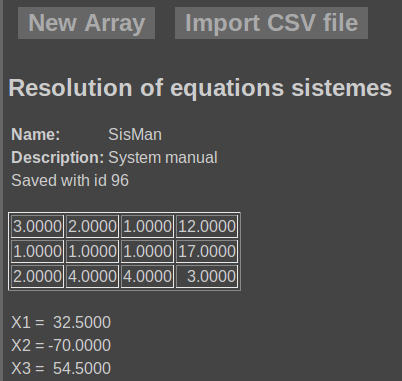If the system is indeterminate would see a window like the figure: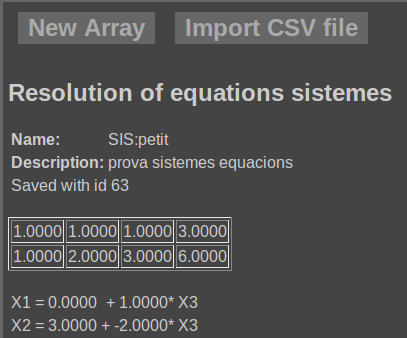# Download a matrix

Also you can download an array with CSV to open it with a spreadsheet. To do so, select the array and combo box select Download. We are presented with a window like the figure: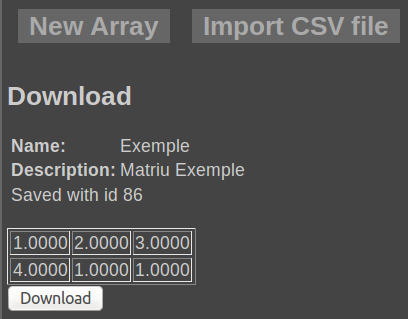Pressing the button to download the CSV file downloaded.